Some basic concepts of chemistry Quiz-36

IIT JEE exam which consists of JEE Main and JEE Advanced is one of the most important entrance exams for engineering aspirants. The exam is held for candidates who are aspiring to pursue a career in the field of engineering and technical studies. Chemistry is important because everything you do is chemistry! Even your body is made of chemicals. Chemical reactions occur when you breathe, eat, or just sit there reading. All matter is made of chemicals, so the importance of chemistry is that it's the study of everything..

Q1.The number of moles of oxygen present in one litre of air under STP conditions (it contains 21% oxygen) is
•  0.246 mol
•  0.07438 mol
•  2.0078 mol
•  0.0094 mol
Solution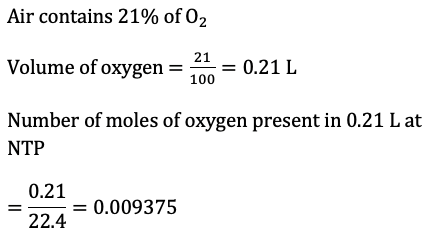Q2.Number of atoms in increasing order in 1.6 g CH_4,1.7 g NH_3 and 1.8 g H_2 O is
•  H_2 O=NH_3=CH_4
•  H_2 O< NH_3< CH_4
•  CH_4< NH_3< H_2 O
•  CH_4=NH_3< H_2 O
Solution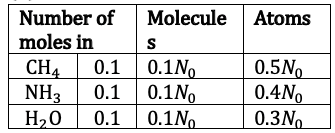Q3. The weight of 1×10^22 molecules of CuSO_4 5H_2 O is
•  4.14 g
•  5.14 g
•  6.14 g
•  7.14 g
Solution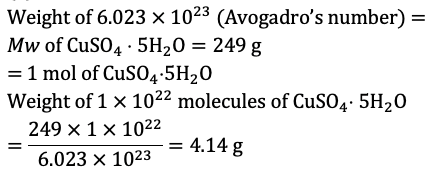Q4. If 10 g of V_2 O_5 is dissolved in acid and is reduced to V^(2+) by zinc metal, how many moles of I_2 could be reduced by the resulting solution if it is further oxidized to VO^(2+) ions? (Atomic mass of V is 51 g mol^(-1))
•  0.11 mol of I_2
•  0.22 mol of I_2
•  0.055 mol of I_2
•  0.44 mol of I_2
Solution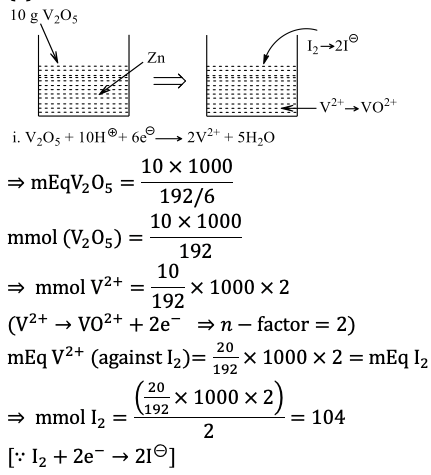Q5. Percentage of nitrogen can be determined by volumetric technique and the method is called
•  Duma’s method
•  Kjeldahl’s method
•  Hofmann’s method
•  Victor’s method
Solution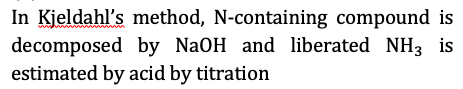Q6. A 5.0 mL solution of H_2 O_2 liberates 1.27 g of iodine from an acidified KI solution, the strength of H_2 O_2 in terms of volume strength is
•  11.2
•  5.6
• 1.7
•  3.4
Solution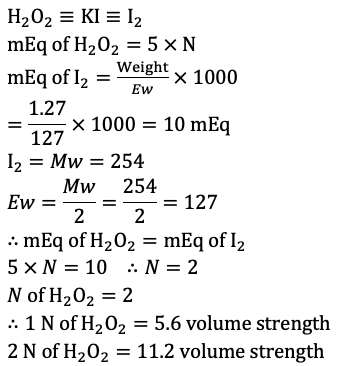Q7.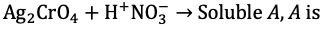•  Ag_2 CrO_4
•  AgNO_3
•  Ag_2 Cr_2 O_7
•  Ag_2 O
Solution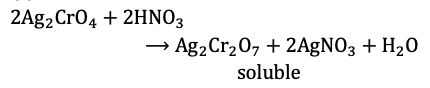Q8. The hydrogen phosphate of certain metal has 〖MHPO〗_4 formula. The formula of metal chloride is
•  MCl
•  M_2 Cl
•  MCl_3
•  MCl_2
Solution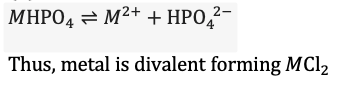Q9. A mixture of Na_2 C_2 O_4 (A)and KHC_2 O_4 .H_2 C_2 O_4 (B) required equal volumes of 0.1 M KMnO_4 and 0.1 M NaOH separated. Molar ratio of A and B in the mixture is
•  1 : 1
•  1 : 5.5
•  5.5 : 1
•  3.1 : 1
Solution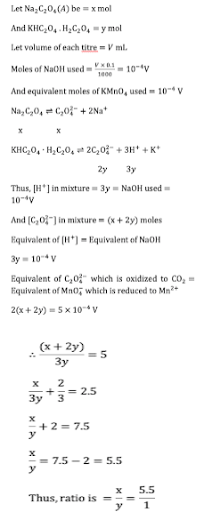Q10. SO_2 can
•  Decolourise MnO_4^-/H^+ solution
•  Turn Cr_2 O_7^(2-)/H^+ solution green
•  Both (a) and (b) are correct
• None of the above is correct
Solution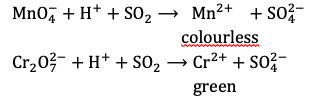Written by: AUTHORNAME

AUTHORDESCRIPTION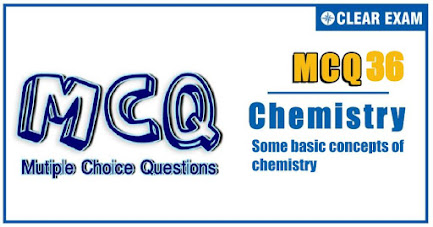Want to know more

Please fill in the details below:

Latest NEET Articles\$type=three\$c=3\$author=hide\$comment=hide\$rm=hide\$date=hide\$snippet=hide

Name

ltr
item
BEST NEET COACHING CENTER | BEST IIT JEE COACHING INSTITUTE | BEST NEET, IIT JEE COACHING INSTITUTE: SOME BASIC CONCEPTS OF CHEMISTRY Quiz-36
SOME BASIC CONCEPTS OF CHEMISTRY Quiz-36